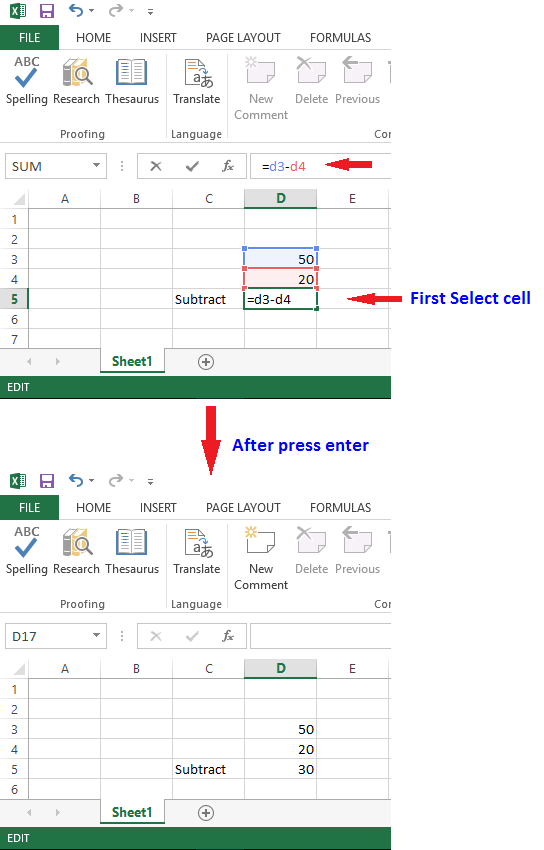# How to do Subtraction in Excel

## How to Subtract Data in Excel

There are two way to Subtract column data in excel first is create formula and other is direct use pre-defined formulas.

### Create Formula

Choose the cell where you want the result to appear as cell D5 in this example. Then type = sign in the cell, now select first cell D3 then type "-" sign then select second cell D4. Now press the enter key, you will get the subtracted data.